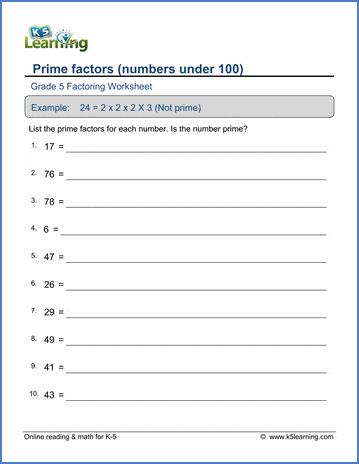# Factoring Worksheets

## Factoring and prime factors worksheets

Our factoring worksheets cover factors, prime factors, greatest common factors (GCFs) and least common multiples (LCMs).  Worksheets of varying difficulty are provided.

• Find the factors of numbers between 4 and 50
• Find the factors of numbers between 4 and 100
• Prime factors & prime factor trees
• Divisibility rules
• Multiples of whole numbers

• Factoring numbers to prime factors (up to 100, 500)
• List all the factors of numbers up to 100
• Prime factor trees
• More divisibility rules.
• Greatest common factor (GCF) of 2 numbers (1-50, 1-100)
• Lowest common multiple (LCM) of 2 numbers 2-30
• GCF and LCM word problems

• Factor numbers up to 500 to prime factors
• Greatest common factor (GCF) of 2 numbers up to 100
• Greatest common factor (GCF) of 3 numbers up to 100
• Least common multiple (LCM) of 2 numbers up to 50
• Least common multiple (LCM) of 3 numbers up to 30

## Related topicsSample Factoring Worksheet

What is K5?

K5 Learning offers free worksheets, flashcards and inexpensive workbooks for kids in kindergarten to grade 5. Become a member to access additional content and skip ads.